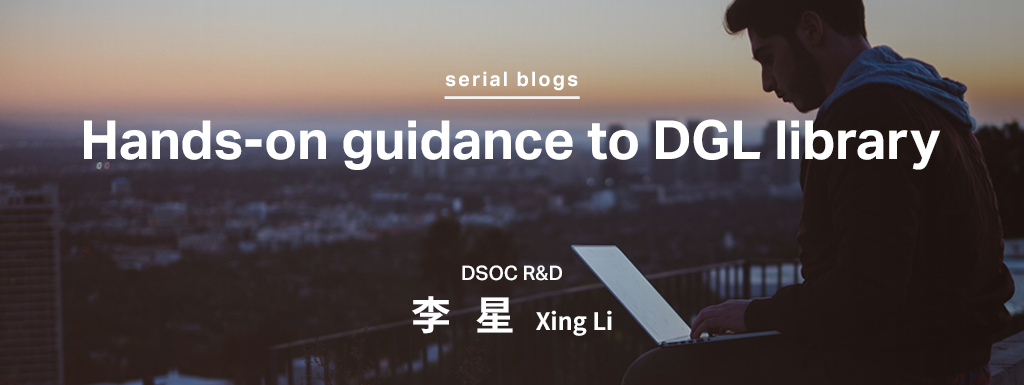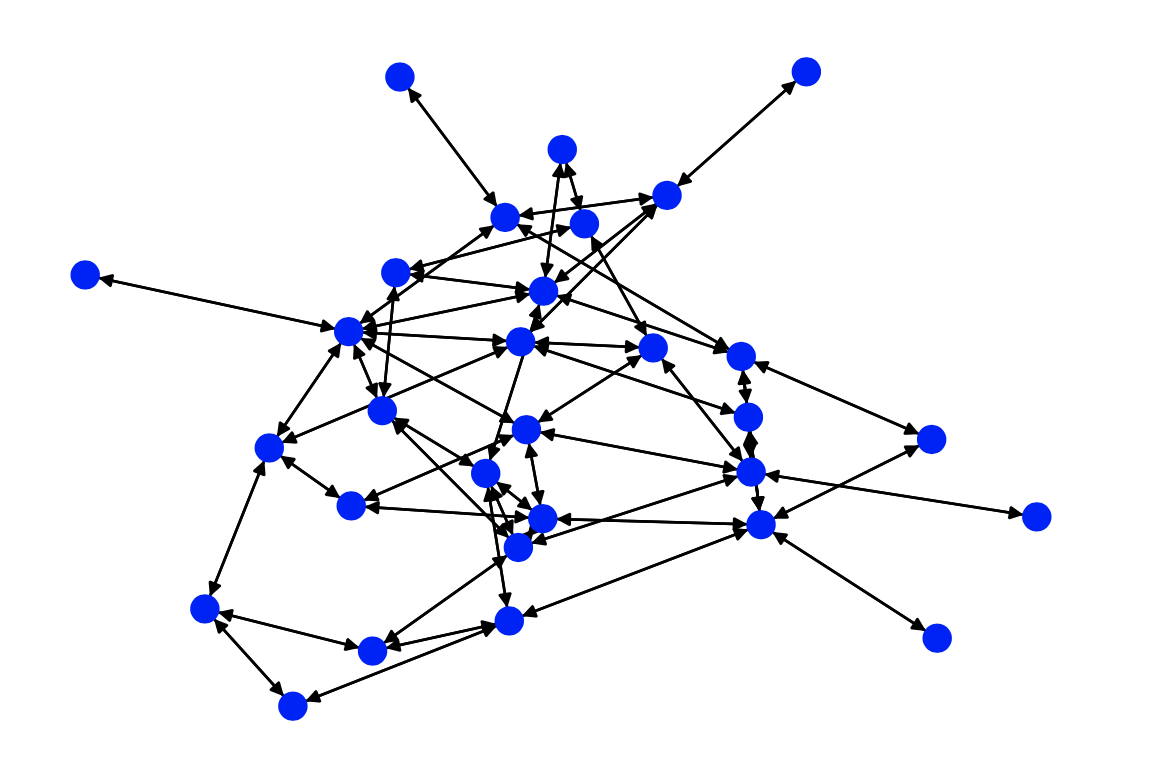# Hands-on guidance to DGL library _ (1) Introduction and Message PassingHi, I am XING LI, a researcher from Sansan DSOC.

Graph is a more general data form to describe our world. Sansan DSOC is creatively exploiting graph data to mining new value for benefitting customers. To coin a phrase, sharp tools make good work. Deep Graph Library(DGL) is just the sharp tool you need to explore deep graph learning. I am planning to post a series hands-on guidance to DGL library together with graph learning topics as examples. This is the first blog of this series, starting with a brief introduction and one core feature of DGL.

# Introduction

In the last few years, many challenging tasks in machine learning field have been extraordinary successfully solved or almost solved by deep learning. These tasks are mainly located in CV(computer vision) or NLP(nature language processing) areas. They share one common feature that most of them are described as euclidean data, the image data usually has a structure of two-dimensional coordinate, sometimes with more layers information and the human language could contain a time series information. While in our real world, non-euclidean data is a more general form of data structure than euclidean data or the tensors. Learning from this more general structured data, more specifically, the graph data, is naturally and widely regarded as an important task.

Recently, Graph Neural Networks(GNNs) family emerges from a tons of models as a versatile fundamental model structure to tackle graph data across several subjects. Such as chemical molecules, social networks, bioinformatics, knowledge graphs and recommendation systems.

An obvious trend in deep learning area is the higher integration of deep learning framework. From Theano, Caffe to current mainstreams Tensorflow, PyTorch. These frameworks provide us an off-the-shelf tool to conveniently and quickly deploy neural networks but also keep the necessary model flexibility for customising specific architectures. In the area of graph neural networks, there are also several frameworks. The two most popular frameworks are Deep Graph Library(DGL) and PyTorch Geometric(PyG).

Name Github Stars Support Team Supported Framework
DGL 4.7K Amazon Web Services PyTorch, TensorFlow and Maxnet
PyG 7.5K TU Dortmund University PyTorch

(All information in this table is retrieved at 12/MAY/2020, DGL 0.4, PyG Latest).

In addition to the above table, DGL could train at most 0.5 billion nodes and 25 billion edges in one single machine with appropriate memory while PyG does not reveal such information. PyG has slightly faster training speed in small graph but DGL is faster when graph is getting larger. Both of them implement most mainstream graph neural network models, but DGL supports more tools and features, such as more optional sampling methods, Heterogeneous Graphs, Knowledge graph and etc..

Here, we only use DGL as the example to explore deep graph learning, but PyG is also a popular framework and worthy of attention. The following contents may involve some concepts of Graph Convolutional Networks and assume other basic understandings of graph learning.

DGL works with the following operating systems:

• Ubuntu 16.04
• macOS X
• Windows 10

Python 3.5 or later version is required to run DGL. More information of installing DGL could be found here.

DGL contains many sophisticated features, such as Message Passing API, Nodeflow Data Structure, Sampling Methods, Heterogeneous Graphs, Scale to Giant Graphs, Knowledge Graph and etc.. They play different roles when facing different requirements and still keep updating continuously. However, some features are fundamental to understand the overall framework and understanding these cornerstones will make it easier to master other features. We will discuss one main feature in this blog: Message Passing.

# Message Passing

Most GNN models perform message-passing style computation over a graph. Such a computation constitutes two main phases, or functions:

• Message function specifies to send a message along an edge from a node to its neighbour. This is edge-wise computation since it is performed for all (or a subset of) edges.
• Reduce function specifies to aggregate the incoming messages of a node and updates the node feature. This is node-wide computation since it is performed for all (or a subset of) nodes.

A classical and typical graph algorithm is PageRanking algorithm.

In each iteration of PageRanking, every node (web page) first scatters its PageRanking value uniformly to its downstream nodes. The new PageRanking value of each node is computed by aggregating the received PageRanking values from its neighbours, which is then adjusted by the damping factor:where N is the number of nodes in the graph; D(v) is the out-degree of a node v andis the neighbour nodes.

To create a DGLGraph, you can flexibly do it from scratch or convert an existing graph from networkx, and vice versa.

Here we create a graph with 30 nodes by using networkx and then convert it to a DGLGraph.

import networkx as nx
import matplotlib.pyplot as plt
import torch
import dgl
%config InlineBackend.figure_format = 'svg'

N = 30  # number of nodes
DAMP = 0.85  # damping factor
K = 10  # number of iterations of message passing

g = nx.nx.erdos_renyi_graph(N, 0.1)
g = dgl.DGLGraph(g) # convert the networkx graph to DGLGraph

nx.draw(g.to_networkx(), node_size=100, node_color=[[0, 0, 1,]])A randomly generated bidirectional graph with 30 nodes

Initialise parameters in PageRanking

g.ndata['pv'] = torch.ones(N) / N # Initialise the PageRanking value of each node to 1/N
g.ndata['deg'] = g.out_degrees(g.nodes()).float() # store each node’s out-degree as a node feature


Define the message function, which divides every node’s PageRanking value by its out-degree and passes the result as message to its neighbours.

def pagerank_message_func(edges):
return {'pv' : edges.src['pv'] / edges.src['deg']}


Define the reduce function, which removes and aggregates the messages from its mailbox, and computes its new PageRanking value.

def pagerank_reduce_func(nodes):
msgs = torch.sum(nodes.mailbox['pv'], dim=1)
pv = (1 - DAMP) / N + DAMP * msgs
return {'pv' : pv}


To understand message passing, my first impression on it is transferred from parallel computing. In parallel computing, each slave thread would independently run identical sub-function and submit their results to the special buffer in master thread. After receiving the notice of finishing from all slave threads, then master thread would run the master function to handle all results submitted in buffer.

This concept could smoothly transfer to graph message passing. The difference is there is no absolute master or slave threads, or nodes here, but all neighbours would be both master nodes and slave nodes. Each node has obligation to submit the message function results to all his neighbours while it also has to handle all message function results from its neighbours.

In DGL, the message functions are expressed as Edge UDFs(user-defined functions). Edge UDFs take in a single argument edge. It has three members src, dst, and data for accessing source node features, destination node features, and edge features. Here in PageRanking, the function computes messages only from source node features.

The reduce functions are Node UDFs. Node UDFs have a single argument node, which has two members data and mailbox. data contains the node features and mailbox contains all incoming message features, stacked along the second dimension.

The message UDF works on a batch of edges, whereas the reduce UDF works on a batch of edges but outputs a batch of nodes. Their relationships are as follows:

Then we need to attach our user-defined message passing functions to a certain DGLGraph, and they could be called later:

g.register_message_func(pagerank_message_func)
g.register_reduce_func(pagerank_reduce_func)


The core algorithm now is simple:

def pagerank_naive(g):
# Phase #1: send out messages along all edges.
for u, v in zip(*g.edges()):
g.send((u, v))
# Phase #2: receive messages to compute new PageRank values.
for v in g.nodes():
g.recv(v)


Run it:

for k in range(K):
pagerank_naive(g)
print(g.ndata['pv'])

# ========== Result==========
tensor([0.0135, 0.0436, 0.0420, 0.0348, 0.0449, 0.0127, 0.0398, 0.0360, 0.0357,
0.0281, 0.0270, 0.0546, 0.0356, 0.0293, 0.0128, 0.0204, 0.0401, 0.0611,
0.0453, 0.0124, 0.0425, 0.0213, 0.0525, 0.0208, 0.0285, 0.0135, 0.0420,
0.0425, 0.0298, 0.0371])


However, the above code does not scale to a large graph because it iterates over all the nodes one by one. DGL solves this by allowing you to compute on a batch of nodes or edges. For example, the following codes trigger message and reduce functions on multiple nodes and edges at one time.

def pagerank_batch(g):
g.send(g.edges())
g.recv(g.nodes())


Till here, if you are also familiar with parallel computing. You might wonder if this is even possible to perform reduce on all nodes in parallel just like what was mentioned above. Since each node may have different number of incoming messages and you cannot really “stack” tensors of different lengths together. In general, DGL solves the problem by grouping the nodes by the number of incoming messages, and calling the reduce function for each group. DGLGraph has already prepared such high-level API:

def pagerank_level2(g):
g.update_all()


Besides, some of the message and reduce functions are used frequently. For this reason, DGL also provides built-in functions for better performance. For example, two built-in functions can be used in the PageRank example.

• dgl.function.copy_src(src, out) - This code example is an edge UDF that computes the output using the source node feature data. To use this, specify the name of the source feature data (src) and the output name (out).
• dgl.function.sum(msg, out) - This code example is a node UDF that sums the messages in the node’s mailbox. To use this, specify the message name (msg) and the output name (out).

And the code is:

import dgl.function as fn

def pagerank_builtin(g):
g.ndata['pv'] = g.ndata['pv'] / g.ndata['deg']
g.update_all(message_func=fn.copy_src(src='pv', out='m'),
reduce_func=fn.sum(msg='m',out='m_sum'))
g.ndata['pv'] = (1 - DAMP) / N + DAMP * g.ndata['m_sum']


All these codes above can produce same output:

for k in range(K):
# pagerank_naive(g)
# pagerank_batch(g)
# pagerank_level2(g)
pagerank_builtin(g)
print(g.ndata['pv'])

# ========== Result==========
tensor([0.0135, 0.0436, 0.0420, 0.0348, 0.0449, 0.0127, 0.0398, 0.0360, 0.0357,
0.0281, 0.0270, 0.0546, 0.0356, 0.0293, 0.0128, 0.0204, 0.0401, 0.0611,
0.0453, 0.0124, 0.0425, 0.0213, 0.0525, 0.0208, 0.0285, 0.0135, 0.0420,
0.0425, 0.0298, 0.0371])


The message passing feature is the core of DGL library. Most graph computation in DGL rely upon it. Play it freely until skilful! We will explore more exhilarating features in future.